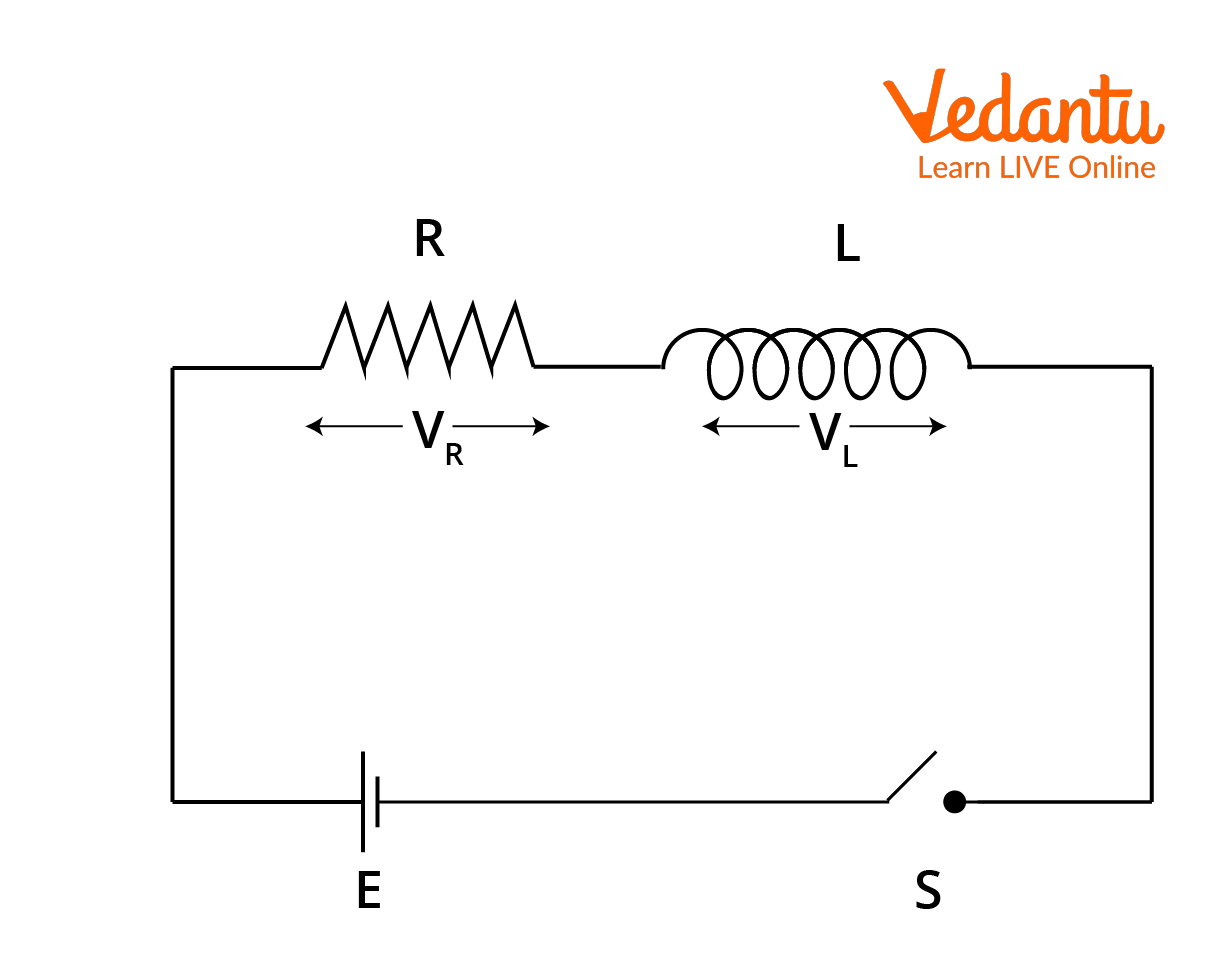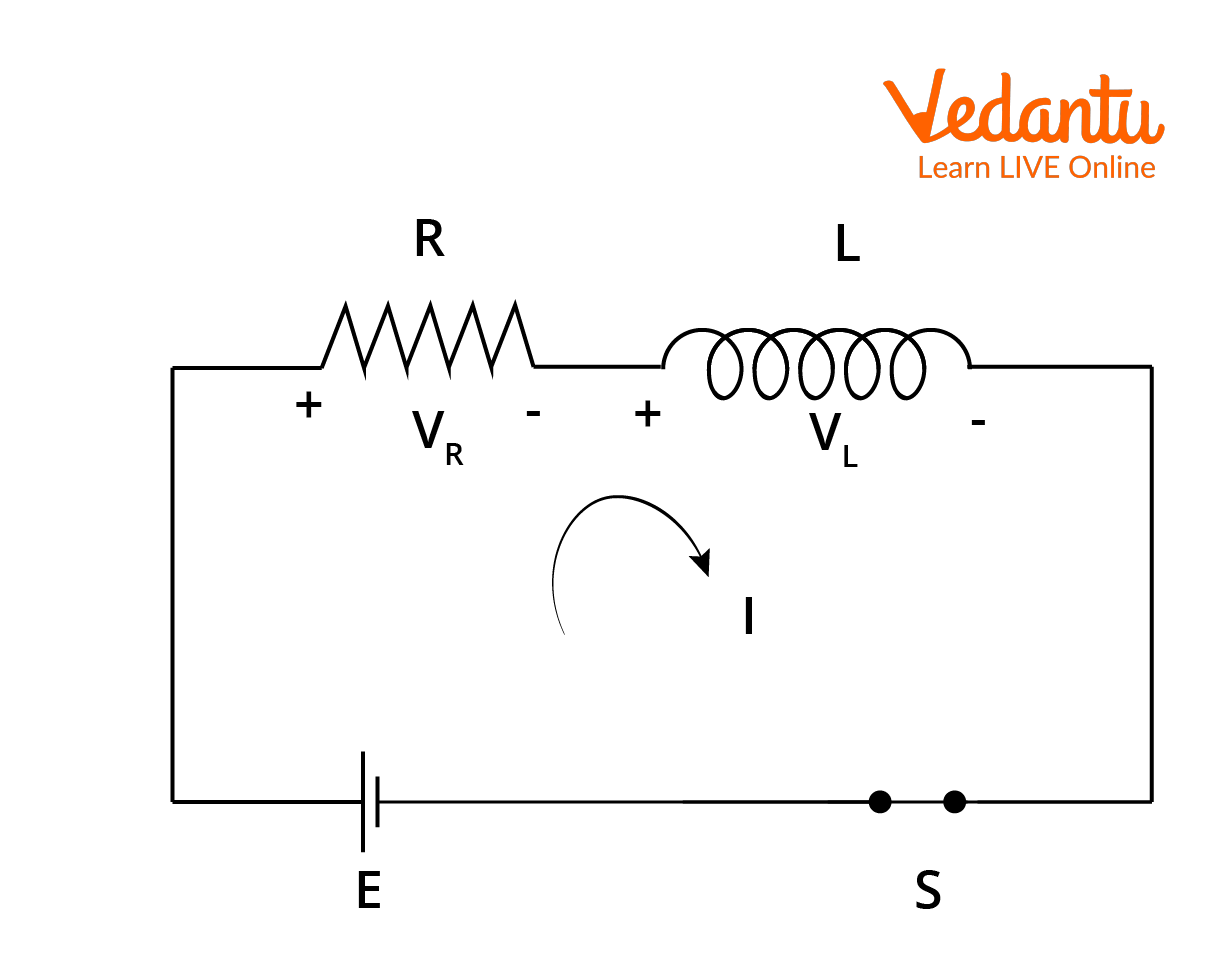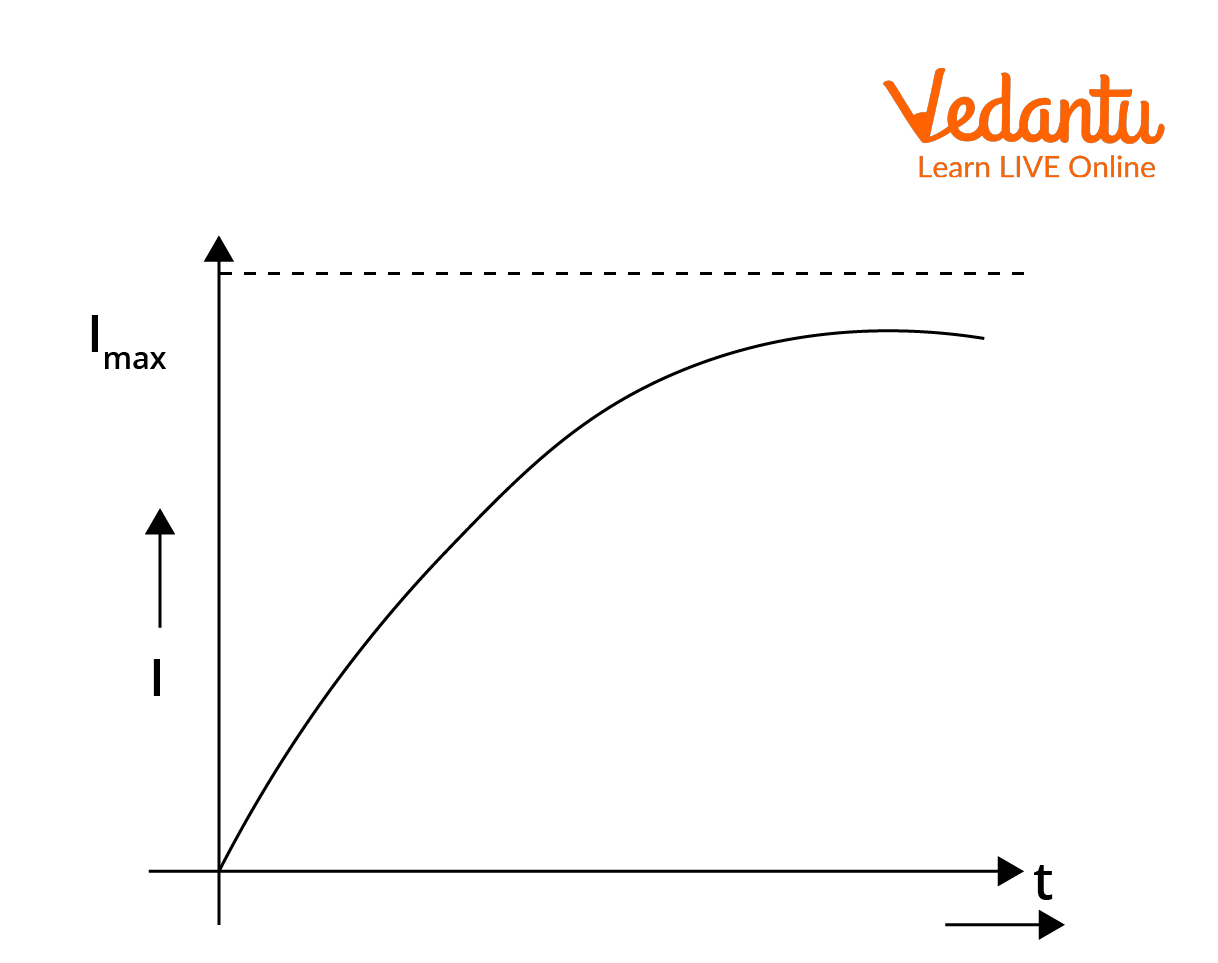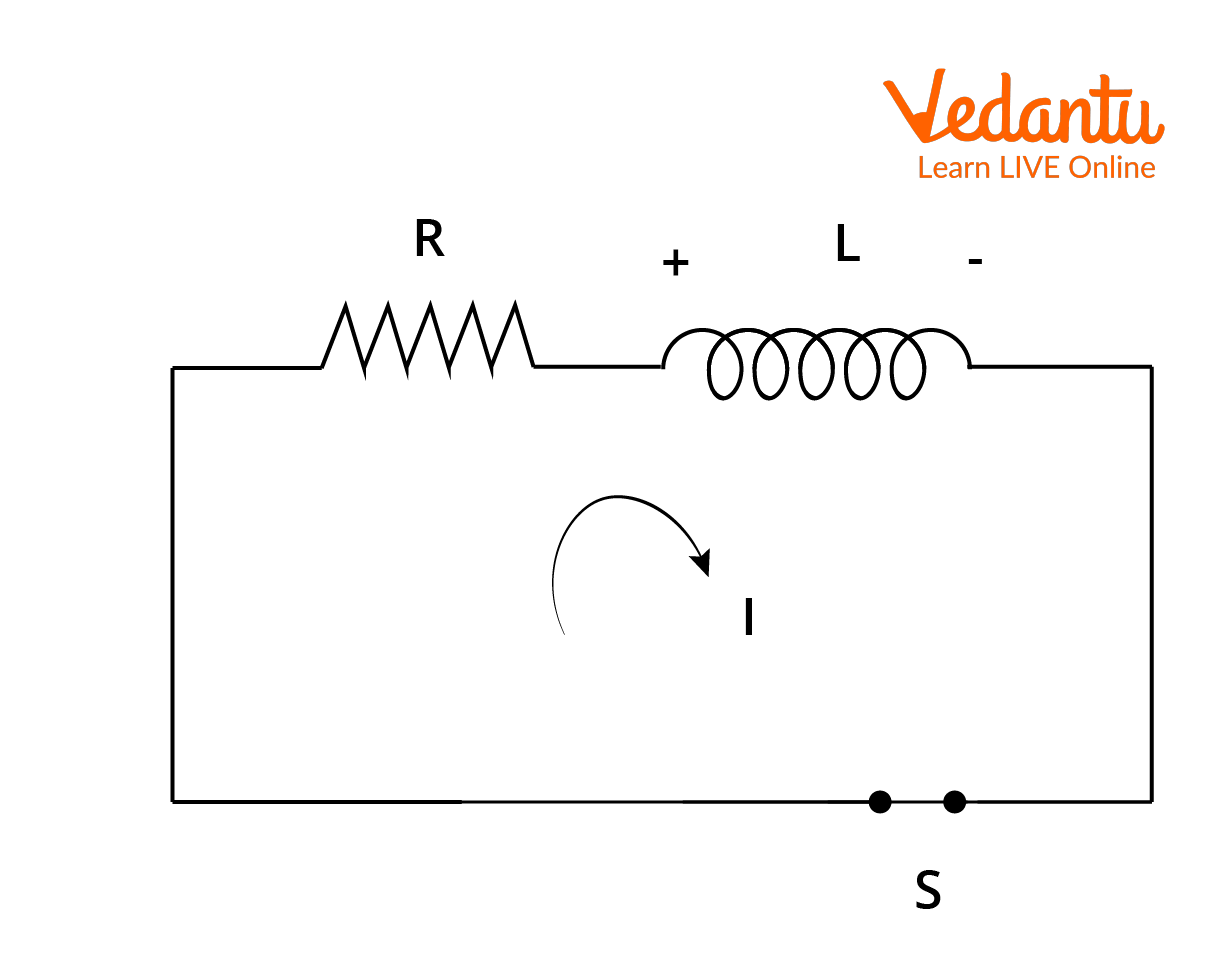Courses
Courses for Kids
Free study material
Free LIVE classes
More

# Growth and Decay of Current in LR Circuits, Formulae and Derivation for JEE MainLIVE
Join Vedantu’s FREE Mastercalss

## Introduction to LR Circuits

Generally, many circuits in electrical and electronics are made up of a combination of resistors, inductors and capacitors. If a circuit is made up of resistors and capacitors, then it is known as an RC circuit. Similarly, when the circuit is containing capacitors and inductors, then it is known as an LC circuit. Therefore, an LR circuit is a circuit which is made up of pure resistors and pure inductors. That means that the circuit is both resistive and inductor and is operated by a voltage source in series or by a current source in parallel.

An LR Circuit is also known as an LR network or LR  filter. When an inductor is connected in series with the resistor, there will be some changes happening in the circuit due to the presence of inductance. The inductor which is present in the circuit opposes the change in magnetic flux, thereby opposing the change in current flowing in the circuit. As we know, according to Faraday's law of magnetic induction, when the current is present in the circuit, there will be a formation of the magnetic field which causes the magnetic flux through the circuit.

When there is any change in the flow of the current, the magnetic flux also changes.  Now,  an EMF  is induced by the variation of the magnetic field around the inductor. Therefore, this EMF is induced by the variation of the own magnetic field of the inductor, so it is known as self-induced EMF. Let us learn how the current in the RL circuit flows and have a look at the LR circuit derivation in detail.

## Growth of Current

An LR Circuit is analysed in three ways. The first one is the initial state, which is present at the instant of closing the switch or opening the switch in the circuit. The second one is the transient state, which appears at any instant after closing or opening the switch. The third one is steady-state, which appears after a long time after closing and opening the switch. Let’s start with the initial and steady states of an LR circuit.

Initial State

Let us assume a circuit of EMF E has the inductance ’L’ and the resistance ‘R’, as shown in the figure. The voltage drop across the inductor is VL and the voltage drop across the resistor is VRInitial Stage of an LR Circuit at t=0

At t = 0, the inductor offers an infinite opposition to the current flow and hence there is no current flow in the circuit at the time of closing the switch. Due to high opposition to the current flow, the voltage is dropped entirely at the inductor and there is no voltage drop across the resistor.

I.e., at t = 0, VR= 0 and VL = E.

At a certain point of time, say t = $\infty$, the current in the inductor does not vary with time after closing or opening the switch for a long period of time. We can see that the current has reached its maximum value and therefore the inductor does not offer any position to the current flow. So, the voltage drop across the inductor becomes zero and the entire voltage drops across a resistor.

At t=$\infty$, VR = E and VL = 0

Transient State

In this state, the voltage is dropped both across the resistor and the inductor. At any instant t = 0 and t = ∞ is taken for this state. We know that the voltage drop across the inductor is equal to the inductance multiplied by the rate of change in current across the inductor.

I.e.,$\mathrm{V}_{\mathrm{L}}=L \dfrac{d I}{d t}$

And the voltage across the resistor is given by VR = IR.

In the transient state, when the switch is closed gradually, the current starts increasing across the inductor. Due to the increase in the current, there will be a self-induced EMF in the inductor which opposes the change of the current in the circuit. Let us take an instant at t = t, the current flowing in the circuit is I as shown in the figure.Transient State of LR Circuit at Time t = t

Let us apply Kirchhoff's voltage law in this circuit.

\begin{align} &E-V_{R}-V_{L}=0 \\ &E-L \dfrac{d I}{d t}- IR=0 \end{align}

Therefore, $\mathrm{E}-\mathrm{IR}=L \dfrac{d l}{d t}$

Taking the integration from t = 0 to t = t and I = 0 to I = I:

\begin{align} &\dfrac{d t}{L}=\dfrac{d I}{E-I R}\\ &\dfrac{1}{L} \int_{0}^{t} d t=\int_{0}^{I} \dfrac{d I}{E-I R}\\ &\dfrac{t}{L}=\left|\dfrac{\ln (E-I R)}{-R}\right|_{0}^{I}\\ &\dfrac{-t R}{L}=(\ln (E-I R)-\ln (E))\\ &\dfrac{-t R}{L}=\ln \dfrac{E-I R}{E}\\&\dfrac{E-I R}{E}=e^{\dfrac{-t R}{L}}\\ &1-\dfrac{I R}{E}=e^{\dfrac{-t R}{L}}\\ &1-e^{\dfrac{-t R}{L}}=\dfrac{I R}{E}\\ &I=\dfrac{E}{R}\left(1-e^{\dfrac{-t R}{L}}\right) \end{align}

Here, $\dfrac{E}{R}$ becomes the maximum current when there is no inductor opposing the current flow. And $\dfrac{L}{R}$ is called the time constant of the LR circuit represented by $\tau$. It is the time for the current to reach 63% of the final current flowing in the circuit. Therefore, putting these values in the equation, we get the final current equation for the growth of the current in the circuit.

$I=I_{0}\left(1-e^{\dfrac{-t}{\tau}}\right)$

This is the instantaneous current at time t = t flowing through the circuit.

Let us draw the graph between current and time and see how the current is increasing with time in the growth of the current state.Graph between Current and Time in the Growth Stage.

Let us similarly derive the current equation in the decaying state of the current.

## Decay of Current

In the Decay of current, the source EMF is removed from the circuit. The current flowing in the circuit will be maximum at the time of this connection of the source. Let maximum current be I0 flowing through the circuit.Decay of Current

At t = 0, the current flowing in the circuit is I0 and at t = t current flowing is I.

By applying Kirchhoff's voltage law and using integration, we obtain:

\begin{align} &-L \dfrac{d I}{d t}-I R=0 \\ &-L \dfrac{d I}{d t}=I R \\ &\dfrac{d I}{I}=-\dfrac{R d t}{L} \\ &\int_{I_{0}}^{I} \dfrac{d I}{I}=-\dfrac{R}{L} \int_{0}^{t} d t \\ &\ln (I)_{I_{0}}^{I}=-\dfrac{R t}{L} \\ &\ln \left(\dfrac{I}{I_{0}}\right)=-\dfrac{t}{\tau} \\ &\dfrac{I}{I_{0}}=e^{-\dfrac{t}{\tau}} \\ &I=I_{0} e^{-\dfrac{t}{\tau}} \end{align}

Therefore, this will be the equation of current decay in the LR circuit.

We derived the LR circuit formula for growth and decay of current in the RL circuit. This LR circuit derivation is very similar in both the cases of growth and decay of current in the LR circuit. Also here, $\dfrac{L}{R}$ is known as the time constant and it is denoted by $\tau$. Let us solve a problem with this concept.

Example: In an LR circuit, when the switch is closed and at an instant t = t, the value of current is 0.1 times the value of maximum current. Given that, the time constant is 5 seconds, find t.

Solution: Given: Current at the instant t = t is 3 times the maximum current i.e. I = 0.1I0  and $\tau$ = 5 sec.

We know that when the switch is closed, the current starts increasing in the circuit. The LR circuit formula for current in growth is given by:

$I=I_{0}\left(1-e^{\dfrac{-t}{\tau}}\right)$

Substituting the given values in the above equation, we get:

\begin{align} &0.1 I_{o}=I_{o}\left(1-e^{\dfrac{-t}{5}}\right) \\ &0.1=\left(1-e^{\dfrac{-t}{5}}\right) \\ &e^{\dfrac{-t}{5}}=0.9 \\ &\dfrac{-t}{5}=\ln (0.9)=-0.105 \\ &t=5 \times 0.105=0.525 \text { seconds } \end{align}

This is the required value of time needed for the current to become 0.1 times the value of the maximum current.

## Conclusion

The LR circuit consists of three stages which are initial, transient and steady states. We have seen how the current in an RL circuit flows with the help of the LR circuit formula and LR circuit derivation. We learned what is the time constant of the LR circuit and how the growth and decay of current in the LR circuit works out.

Last updated date: 17th Sep 2023
Total views: 132.9k
Views today: 1.32k

## FAQs on Growth and Decay of Current in LR Circuits, Formulae and Derivation for JEE Main

1. What are the three different stages of current flow and analysis in an LR circuit?

There are three different stages in which the LR Circuit is analysed. These are the initial state, transient state, and steady-state. In the initial state, the current increases across the inductor, and the inductor offers a large opposition to the current flow at the instant of closing the switch. Whereas in the transient state, there is a voltage drop across the inductor and resistor at any instant of time. In the steady state, the current attains its maximum value, and thereby the inductor will not produce any opposition to the current flow. In this steady state, the entire voltage drops across the resistor.

2. What is the weightage of this topic in JEE?

The topic of LR circuits is very important in the JEE examination. Generally in every exam, a minimum of one question from LR circuits, LC circuits, or RC circuits will be asked. This concept is the basis of any wide variety of concepts in AC circuits. The topic of growth and decay of current in the LR circuit and the formulae are very important if we want to find the current flowing in the circuit, consisting of resistors and inductors at a certain point of time, when the switch is closed or opened.